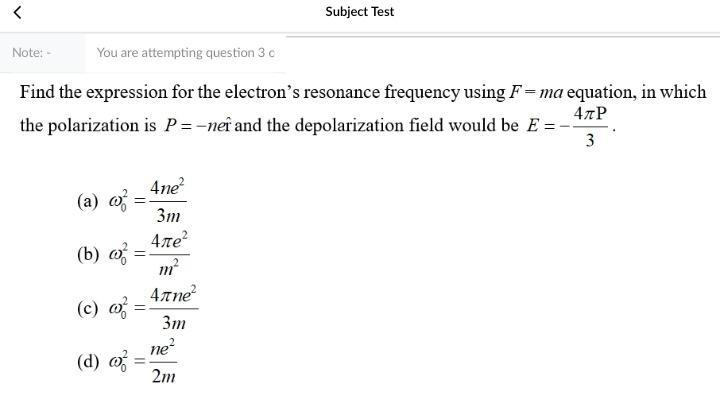Question:

# < Subject Test Note: You are attempting question 3 Find the expression for the electron's resonance frequency using F=ma equatio< Subject Test Note: You are attempting question 3 Find the expression for the electron's resonance frequency using F=ma equation, in which 40P the polarization is P=-neî and the depolarization field would be E = 3 (a) 02 (b) 03 = 4ne? 3m 41e? m? 41 ne? 3m ne? 2m (c) = (d) o